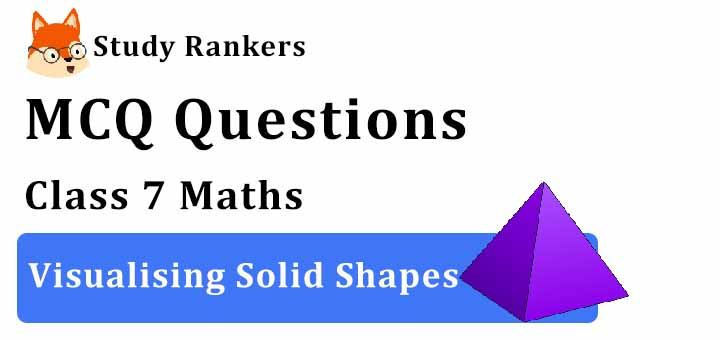## MCQ Questions for Class 7 Maths: Ch 15 Visualising Solid Shapes1. The vertical cut of a brick will show the cross section is
(a) circle
(b) pentagon
(c) rectangle
(d) hexagon
► (c) rectangle

2. The number of faces of a triangular prism is _______.
(a) 5
(b) 6
(c) 4
(d) None of these
► (c) 4

3. The number of faces of a cube is _______.
(a) 4
(b) 6
(c) 8
(d) None of these
► (b) 6

4. A cuboid has ________ rectangular faces.
(a) 8
(b) 2
(c) 6
(d) 4
► (c) 6

5. The number of faces of a cylinder is _______.
(a) 2
(b) 1
(c) 3
(d) None of these
► (a) 2

6. The number of faces of a square pyramid is _______.
(a) 4
(b) 7
(c) 5
(d) None of these
► (c) 5

7. Identify the correct statement from the following.
(a) A triangle has 3 sides and 4 vertices.
(b) A cylinder has 3 faces.
(c) All sides of the rectangle are equal.
(d) A cuboid has 4 flat faces and 12 straight edges.
► (b) A cylinder has 3 faces.

8. The number of faces of a rectangular prism is _______.
(a) 4
(b) 6
(c) 3
(d) None of these
► (b) 6

9. The number of edges of a square pyramid is _______.
(a) 4
(b) 6
(c) 8
(d) None of these
► (c) 8

10. The number of edges of a rectangular pyramid is _______.
(a) 21
(b) 8
(c) 7
(d) None of these
► (b) 8

11. The number of edges of a triangular pyramid is _______.
(a) 8
(b) 5
(c) 6
(d) None of these
► (c) 6

12. The number of faces of a triangular pyramid or tetrahedron is _______.
(a) 4
(b) 6
(c) 5
(d) 1024
► (a) 4

13. The number of triangular faces of a triangular prism is _______.
(a) 2
(b) 1
(c) 4
(d) None of these
► (a) 2

14. Cuboid is an example of
(a) Both
(b) 2-D shape
(c) 3-D shape
(d) None of these
► (c) 3-D shape

15. The number of vertices of a cube is _______.
(a) 12
(b) 8
(c) 6
(d) None of these
► (b) 8

16. Which of the following is the number of vertices of sphere?
(a) 0
(b) 1
(c) 2
(d) 4
► (a) 0

17. A die is cut horizontally. What is the cross-section obtained?
(a) A triangle
(b) A rectangle
(c) A square
(d) A cube
► (c) A square

18. Rakesh has 10 one rupee coins of similar kind. He puts them exactly one on the other. What shape will he get finally?
(a) Circle
(b) Cylinder
(c) Cube
(d) Cone
► (b) Cylinder